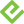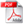# Microsoft Excel 2013 Data Analysis and Business Modeling

## Best Value Purchase

### Book + eBook Bundle

• List Price: \$89.98
• Includes EPUB, MOBI, and PDF
• This eBook includes the following formats, accessible from your Account page after purchase:EPUB The open industry format known for its reflowable content and usability on supported mobile devices.MOBI The eBook format compatible with the Amazon Kindle and Amazon Kindle applications.PDF The popular standard, used most often with the free Adobe® Reader® software.

This eBook requires no passwords or activation to read. We customize your eBook by discreetly watermarking it with your name, making it uniquely yours.

## More Purchase Options

### Book

• List Price: \$49.99
• Usually ships in 24 hours.

### eBook (Watermarked)

• List Price: \$39.99
• Includes EPUB, MOBI, and PDF
• This eBook includes the following formats, accessible from your Account page after purchase:EPUB The open industry format known for its reflowable content and usability on supported mobile devices.MOBI The eBook format compatible with the Amazon Kindle and Amazon Kindle applications.PDF The popular standard, used most often with the free Adobe® Reader® software.

This eBook requires no passwords or activation to read. We customize your eBook by discreetly watermarking it with your name, making it uniquely yours.

### Features

• Helps students master advanced Excel functions such as OFFSET and INDIRECT
• Applies important modeling tools such as the Inquire add-in
• Covers new trends in predictive and prescriptive analytics
• Teaches Excel Trend Curves, multiple regression, and exponential smoothing
• Delves into key financial, statistical, and time functions

## Description

• Dimensions: 7-3/8" x 9"
• Pages: 896
• Edition: 1st
• Book
• ISBN-10: 0-7356-6913-9
• ISBN-13: 978-0-7356-6913-0

Master business modeling and analysis techniques with Microsoft Excel 2013, and transform data into bottom-line results. Written by award-winning educator Wayne Winston, this hands-on, scenario-focused guide shows you how to use the latest Excel tools to integrate data from multiple tables—and how to effectively build a relational data source inside an Excel workbook.

• Summarize data with PivotTables and Descriptive Statistics
• Explore new trends in predictive and prescriptive analytics
• Use Excel Trend Curves, multiple regression, and exponential smoothing
• Master advanced Excel functions such as OFFSET and INDIRECT
• Delve into key financial, statistical, and time functions
• Make your charts more effective with the Power View tool
• Tame complex optimization problems with Excel Solver
• Run Monte Carlo simulations on stock prices and bidding models
• Apply important modeling tools such as the Inquire add-in

2. If prompted, click Save.
3. Locate the .zip file on your computer. Right-click the file, click Extract All, and then follow the instructions.

## Sample Content

### Sample Pages

• Introduction
• Chapter 1: Range names
• Chapter 2: Lookup functions
• Chapter 3: INDEX function
• Chapter 4: MATCH function
• Chapter 5: Text functions
• Chapter 6: Dates and date functions
• Chapter 7: Evaluating investments by using net present value criteria
• Chapter 8: Internal rate of return
• Chapter 9: More Excel financial functions
• Chapter 10: Circular references
• Chapter 11: IF statements
• Chapter 12: Time and time functions
• Chapter 13: The Paste Special command
• Chapter 14: Three-dimensional formulas
• Chapter 15: The Auditing tool and Inquire add-in
• Chapter 16: Sensitivity analysis with data tables
• Chapter 17: The Goal Seek command
• Chapter 18: Using the Scenario Manager for sensitivity analysis
• Chapter 19: The COUNTIF, COUNTIFS, COUNT, COUNTA, and COUNTBLANK functions
• Chapter 20: The SUMIF, AVERAGEIF, SUMIFS, and AVERAGEIFS functions
• Chapter 21: The OFFSET function
• Chapter 22: The INDIRECT function
• Chapter 23: Conditional formatting
• Chapter 24: Sorting in Excel
• Chapter 25: Tables
• Chapter 26: Spinner buttons, scroll bars, option buttons, check boxes, combo boxes, and group list boxes
• Chapter 27: The analytics revolution
• Chapter 28: Introducing optimization with Excel Solver
• Chapter 29: Using Solver to determine the optimal product mix
• Chapter 30: Using Solver to schedule your workforce
• Chapter 31: Using Solver to solve transportation or distribution problems
• Chapter 32: Using Solver for capital budgeting
• Chapter 33: Using Solver for financial planning
• Chapter 34: Using Solver to rate sports teams
• Chapter 35: Warehouse location and the GRG Multistart and Evolutionary Solver engines
• Chapter 36: Penalties and the Evolutionary Solver
• Chapter 37: The traveling salesperson problem
• Chapter 38: Importing data from a text file or document
• Chapter 39: Importing data from the Internet
• Chapter 40: Validating data
• Chapter 41: Summarizing data by using histograms
• Chapter 42: Summarizing data by using descriptive statistics
• Chapter 43: Using PivotTables and slicers to describe data
• Chapter 44: The Data Model
• Chapter 45: PowerPivot
• Chapter 46: Power View
• Chapter 47: Sparklines
• Chapter 48: Summarizing data with database statistical functions
• Chapter 49: Filtering data and removing duplicates
• Chapter 50: Consolidating data
• Chapter 51: Creating subtotals
• Chapter 52: Charting tricks
• Chapter 53: Estimating straight-line relationships
• Chapter 54: Modeling exponential growth
• Chapter 55: The power curve
• Chapter 56: Using correlations to summarize relationships
• Chapter 57: Introduction to multiple regression
• Chapter 58: Incorporating qualitative factors into multiple regression
• Chapter 59: Modeling nonlinearities and interactions
• Chapter 60: Analysis of variance: one-way ANOVA
• Chapter 61: Randomized blocks and two-way ANOVA
• Chapter 62: Using moving averages to understand time series
• Chapter 63: Winters's method
• Chapter 64: Ratio-to-moving-average forecast method
• Chapter 65: Forecasting in the presence of special events
• Chapter 66: An introduction to random variables
• Chapter 67: The binomial, hypergeometric, and negative binomial random variables
• Chapter 68: The Poisson and exponential random variable
• Chapter 69: The normal random variable
• Chapter 70: Weibull and beta distributions: modeling machine life and duration of a project
• Chapter 71: Making probability statements from forecasts
• Chapter 72: Using the lognormal random variable to model stock prices
• Chapter 73: Introduction to Monte Carlo simulation
• Chapter 74: Calculating an optimal bid
• Chapter 75: Simulating stock prices and asset allocation modeling
• Chapter 76: Fun and games: simulating gambling and sporting event probabilities
• Chapter 77: Using resampling to analyze data
• Chapter 78: Pricing stock options
• Chapter 79: Determining customer value
• Chapter 80: The economic order quantity inventory model
• Chapter 81: Inventory modeling with uncertain demand
• Chapter 82: Queuing theory: the mathematics of waiting in line
• Chapter 83: Estimating a demand curve
• Chapter 84: Pricing products by using tie-ins
• Chapter 85: Pricing products by using subjectively determined demand
• Chapter 86: Nonlinear pricing
• Chapter 87: Array formulas and functions